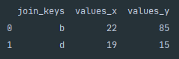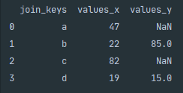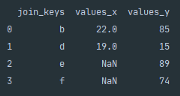## Merging Pandas DataFrames in Python

[ad_1]

By Dean McGrath, Aspiring Data Analyst### Introduction

As Data Scientist, we will often find that we are required to analyse data from multiple data sources at the one time. To be successful at achieving this, we need to be able to merge different data sources using a variety of methods efficiently. Today we are going to look at using Pandas built-in `.merge()` function to join two data sources using several different join methods.

### Getting Started

For those of you that are new to data science or haven’t been exposed to Python Pandas yet, we recommend first beginning with Pandas Series & DataFrame Explained or Python Pandas Iterating a DataFrame. Both of these articles will provide you with the installation instructions and background knowledge for today’s article.

### Pandas Merge

The Pandas built-in function `.merge()` provides a powerful method for joining two DataFrames using database-style joins.

Syntax

The above Python snippet shows the syntax for Pandas .merge() function.

### Parameters

• `right` — This will be the DataFrame that you are joining.
• `how` — Here, you can specify how you would like the two DataFrames to join. The default is `inner` however, you can pass `left` for left outer join, `right` for right outer join and `outer` for a full outer join.
• `on` — If both DataFrames contain a shared column or set of columns, then you can pass these to `on` as keys to merge.
• `left_on` — Here, you can specify a column or list of labels that you would like to join the left DataFrame. This parameter is handy when the columns that you would like to join on in both DataFrames are named differently.
• `right_on` — Same conditions apply as with `left_on` however, for the right DataFrame.
• `left_index` — If you would like to join the left DataFrame using the index, then pass `True`.
• `right_index` — If you would like to join the right DataFramse using the index, then pass `True.`
• `sort` — Here you can supply `True` if you would like the joined keys sorted in lexicographical order.
• `suffixes` — If the two DataFrames share column label names, then you can specify the type of suffixes to apply to the overlapping. The default is `_x` for left and `_y` for right.
• `indicator` — You can set this parameter to `True` if you would like to flag the source of the row. This flag will indicate whether the rows keys appeared in the left DataFrame only, the right DataFrame or in both DataFrames.
• `validate` — Here, you can check to see how the DataFrames joined and the relationship between the keys. You can pass the following `1:1` to check if the keys are unique in the left and right DataFrames, `1:m` to check if the merged keys are unique to the left DataFrame only, `m:1` to check if the merged keys are unique to the right DataFrame only.

### Practical Application

Below we are going to work through several examples of how you can use the merge function yourself. The snippet provided below will assist you in creating two DataFrames that we will be using in the remainder of the story.

The above Python snippet creates two DataFrames that you can use to follow on with the below examples.The above console output shows the result of executing the Python snippet to create two DataFrames.

### Inner Join

The inner join method is Pandas merge default. When you pass `how='inner'` the returned DataFrame is only going to contain the values from the joined columns that are common between both DataFrames.

The above Python snippet demonstrates how to join the two DataFrames using an inner join.The above image shows the console output after inner joining the two DataFrames.

From the above screenshot of the console output, we can see the effects of the inner join on the two DataFrames. As values `a`, `c`, `e` and `f` are not shared across the two DataFrames they do not appear in the console output. The output additionally demonstrates the default suffix application when dealing with a shared column label across two DataFrames.

### Left Join

Pandas left join functions in a similar way to the left outer join within SQL. The returned DataFrame is going to contain all the values from the left DataFrame and any value that matches a joining key during the merge from the right DataFrame.

The above Python snippet shows the syntax for merging the two DataFrames using a left join.The above screenshot shows the console output for merging the two DataFrames using a left join.

If the right DataFrame does not match a value within the merged column `NaN`Not a Number, will be inserted in the returned DataFrame.

### Right Join

Pandas right join performs a similar function to the left join however the join method is applied to the Right DataFrame.

The above Python snippet shows the syntax for merging the two DataFrames using Pandas right join.The above screenshot shows the result of merging the two DataFrames using the right join.

As seen above, any key that does not exist from the right, in the left DataFrame, will have a `NaN` value inserted.

### Outer Join

Pandas outer join merges both DataFrames and essentially reflects the outcome of combining a left and right outer join. The outer join will return all values from both the left and right DataFrame. Where Pandas cannot find a value within the merging DataFrame `NaN` will be used in place.

The above Python snippet shows the syntax for joining the two DataFrames using an outer join.The above screenshot shows the result of merging the two DataFrames using an outer join.

When merging two DataFrames using an outer join, it is sometimes useful information to know where the record within the new DataFrame originated. You can view the origin of a record by passing `indicator=True` as a parameter to the `.merge()` function which will create a new column titled `_merge`.

### Summary

To be successful as a Data Scientist, you need to be skilled in handling data from multiple data sources often at the same time. Frequently we will need to combine data sources sometimes to enrich a dataset or merge historical snapshots within current data. Pandas provide a powerful method for joining dataset using the built-in `.merge()` function. Pandas `.merge()` function offers flexibility in the types of joins that you can create to achieve the desired output.

Thank you for taking the time to read our story — we hope you have found it valuable!

Bio: Dean McGrath (@DeanMcGrath8) is an Aspiring Data Analyst, and contributor to Towards Data Science.

Original. Reposted with permission.

Related:

Read More …

[ad_2]